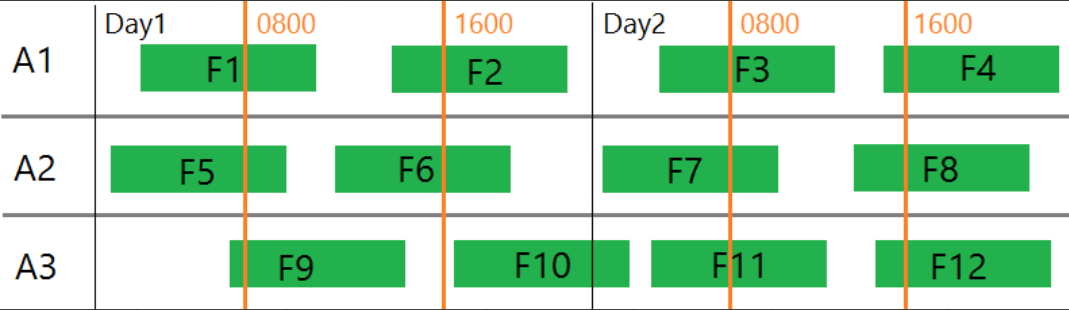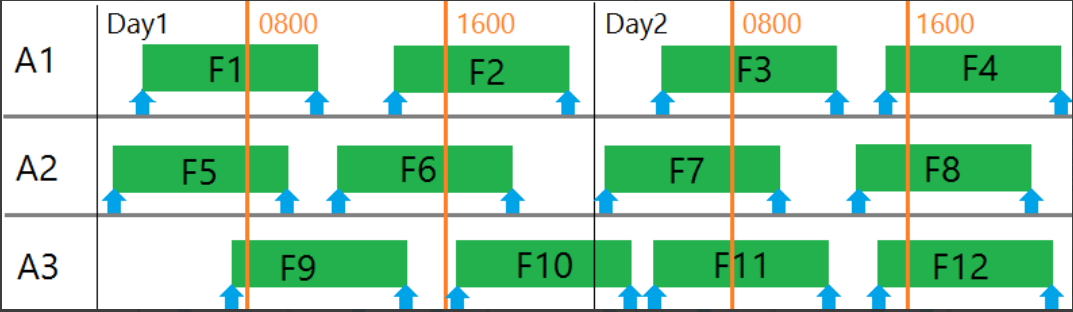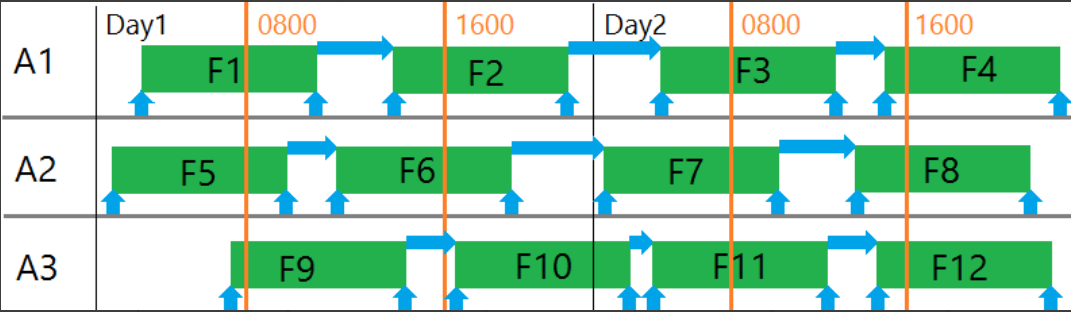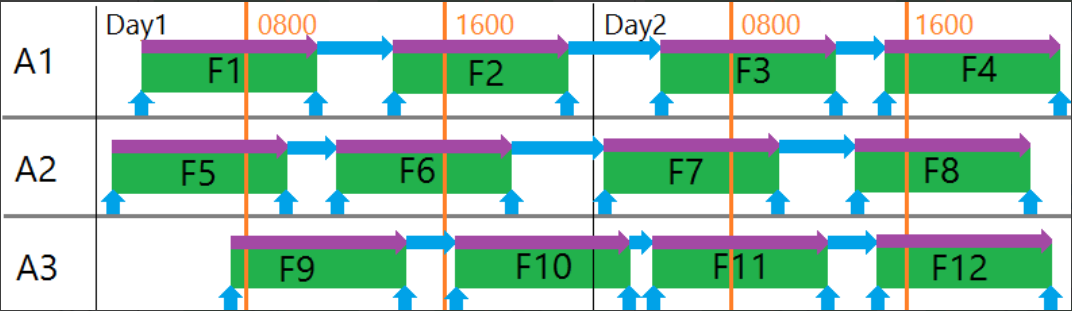# The Idea behind Cost calculation

Cost calculation is based on:
• legs (and indirectly on aircrafts),
• costs definitions
• cost formula
Cost calculation algorithm take into account so called "events" that are generated from selected legs grouped by aircrafts the legs are assigned to.
Let's assume following example with 3 aircrafts (Aircraft A1, Aircraft A2, Aircraft A3),
12 flights (Flight F1, Flight F2, ...., Flight F12) within 2 following Days (Day1 and Day2) in different time (0800h and 1600h are depicted with orange lines)Each such flight can be transformed into special (internally defined in the system (hardcoded)) events.
In the picture below they are depicted as blue arrows. We can easily define such events as TakeOff and Landing
that are shown as an arrow at the beginning and at the end of a flight (this kind of event is a point in time).Additionally we can imagine that between Landing event and Take Off event there is a Handling event that is a period in time -
has its beginning and end and some distinct point in time that defines such event as a point instead of a period.
Please note the horizontal blue arrows between flights.We can also imagine the event that is flight itself (for example fuel burnt by a plane corresponds to the time of flight) - in the picture below please note violet arrows above each flight.Cost algorithm takes into account only this kind of events (the arrows shown in the pictures above).
All events are grouped by an aircraft final cost is calculated based on definitions configuration and chosen definitions selected as an input for the algorithm.

 Example 1 from 0000 till 0800 (exclusive) Landing fee can be equal to 50EURfrom 0800 (inclusive) till 1600 Landing fee can be equal to 100EURfrom 1600 (inclusive) till 2400 (exclusive) Landing fee can be equal to 75EUR Example 2 from 0800 till 1600 Landing fee can be equal to 50EURfrom 1600 till 0800 Landing fee can be equal to 100EUR

If a definition is a period (i.e. Handling) then the cost of such event is calculated in parts to conform to the cost that can change in time.
 Example: If Handling is different between 0800-1600 and 1600-0800 then the cost will be calculated appropriately. In case of flights F9 and F10 the cost will be calculated differentlyfrom landing till 1600 (exclusive)and from 1600 (inclusive) till take off.Same thing can happen when a day/month/year/day of week changes.

Cost can be dependent to vehicle type.

 Example: If aircraft A1 is Boeing 737 with Maximum Take Off Weight equal to X,and aircraft A2 can be Airbus A380 with MTOW equal to Ythen they can have different costs of landing/take off on the same airportand this different costs will be taken into account by the calculation algorithm.

Cost for each airport can be in different currency (i.e. MMX, STO in SEK, LHR in GBP, WAW in PLN) this can be defined in a cost definition and based on the exchange currency table they will be recalculated into 1 result currency i.e. EUR.

Inputs:
Algorithm inputs are:
• definitions (that defines important cost base data) with
so called sets (that defines grouping/category for example per airport i.e. definition=Landing, Filter=CPH airport)

and set values (that defines validity periods (from-to), hours validity, frequency, additional filters (dependent on event "type")
and cost i.e. from 01JAN17 till 31DEC17 from Monday till Friday from 0800 till 1600 price is 100 EUR).

• formula
• currency scenario (actually based static exchange rate tables)
• result currency
Output:
Algorithm output is value in selected result currency grouped by selected currency scenarios.Overview:

 Example: Let's assume following situation: There are 4 flights:2 flights for aircraft A1 of type Boeing 737     Flight F1 from MMX to WAW, STD=1200, STA=1315and      Flight F2 from WAW to FRA, STD=1700, STA=1900 2 flights for aircraft A2 of type Airbus A380     Flight F3 from MMX to WAW, STD=0600, STA=0710and     Flight F4 from WAW to FRA, STD=0900, STA=1100 There are following costs definitions: Name: TakeOff, per eventMMX, 0000-0000 (24h), 500 SEK for 7370000-0000 (24h), 1000 SEK for A380WAW0800-1600, 100 PLN for 7371600-0800, 200 PLN for 7370800-1600, 150 PLN for A3800800-1600, 300 PLN for A3800000-0000 (24h), 50 PLN (for any other type)FRA0800-1600, 100 EUR (for any type of aircraft)1600-0800, 200 EUR (for any type of aircraft) Name: Landing, per eventWAW0800-1600, 50 PLN for 7371600-0800, 75 PLN for 7370800-1600, 100 PLN for A3800800-1600, 125 PLN for A3800000-0000 (24h), 25 PLN (for any other type)FRA0000-0000 (24h), 50 EUR (for any type fo aircraft) Name: Handling, per minuteMMX0800-1600, 10 SEK1600-0800, 20 SEKWAW0800-1600, 5 PLN1600-0800, 10 PLN for 7371600-0800, 15 PLN for A380FRA1000-2000, 10 EUR2000-1000, 15 EUR There are following formula definitions Name: Formula 1, Expression: 2 * Landing + Handling + 3 * TakeOff There are following Exchange rate tables defined Name: Expected, Rates:1 EUR = 4 PLN1 PLN = 0.5 SEK1 EUR = 9 SEK User executes cost calculation algorithm with all above selected and result currency set to PLN, so: flights: F1, F2, F3, F4Definitions: TakeOff, Handling, LandingFormula: Formula 1Currency scenario: ExpectedResult currency: PLN

Steps of the algorithm:

1. transforms selected flights into events - following events will be created:

• event E1: TakeOff for flight F1 (MMX, 737, STD=1200)
• event E2: Landing for flight F1 (WAW, 737, STA=1315)
• event E3: TakeOff for flight F2 (WAW, 737, STD=1700)
• event E4: Landing for flight F2 (FRA, 737, STA=1900)
• event E5: Handling for aircraft A1 between flight F1 and F2 (WAW, 737, 1315-1700)
• event E6: TakeOff for flight F3 (MMX, A380, STD=0600)
• event E7: Landing for flight F3 (WAW, A380, STA=0710)
• event E8: TakeOff for flight F4 (WAW, A380, STD=0900)
• event E9: Landing for flight F5 (FRA, A380, STA=1100)
• event E10: Handling for aircraft A2 between flight F3 and F4 (WAW, A380, 0710-0900)

2. Selected formula "2 * Landing + Handling + 3 * TakeOff" will be "tokenizen" (itemized) and calculation will be done for each definition "token"

a. first - processing will be done for Landing token - appropriate definition will be searched in the definitions given as input to the engine and because there is such a definition name equal to "Landing" then
•             all Lading events will be processed in this step (so events E2, E4, E7 and E9)
•             for each of those events appropriate value will be calculated (E2 (WAW+737)=50 PLN, E4 (FRA+737)=50 EUR, E7 (WAW+A380)=100 PLN, E9 (FRA+A380)=50 EUR) so the cost for Landing is "150PLN + 100 EUR")
•             this value (150PLN + 100 EUR) will be put instead of Landing definition token in the processed formula

b. formula looks like this: "2 * (150PLN + 100EUR) + Handling + 3 * TakeOff"

c. second - processing of Handling token will be done - appropriate definition will be searched in the definitions given as input to the engine and because there is such a definition name equal to "Handling" then
•               all Handling events will be processed in this step (so events E5 and E10)
•               for each of those events appropriate value will be calculated (E5 - will be divided into parts 1315-1600 (165 minutes * 5 PLN=825PLN)
and 1600-1700 (60 minutes * 10 PLN=600PLN) overall sum = 1425 PLN; E10 - will be    divided into parts too: 0710-0800 (50 minutes * 15PLN=750PLN)
and 0800-0900 (60 minutes * 5PLN=300PLN) overall sum = 1050PLN) so the cost for Handling is "2475 PLN"
•               this value (2475PLN) will be put instead of Handling definition token in the processed formula

d. formula looks like this: "2 * (150PLN + 100EUR) + (2475PLN) + 3 * TakeOff"

e. third - processing of TakeOff token will be done - appropriate definition will be searched in the definitions given as input to the engine and because there is such a definition name equal to "TakeOff" then
•               all TakeOff events will be processed in this step (so events E1, E3, E6, E8)
•               for each of those events appropriate value will be calculated (E1 (MMX+737)=500 SEK, E3 (WAW+737+1700)=200 PLN,
E6 (MMX+A380)=1000 SEK, E8 (WAW+A380+0900)=300 PLN) so the cost for TakeOff is "1500 SEK + 500PLN"
•              this value (1500 SEK + 500PLN) will be put instead of TakeOff definition token in the processed formula

f. formula looks like this: "2 * (150PLN + 100EUR) + (2475PLN) + 3 * (1500 SEK + 500PLN)"

If there will be a landing in MMX of an aircraft type Boeing 737 then the cost of such Landing will be set to (MMX+737)=0 PLN
(when there is no fee then result currency is taken into account and the cost taken into further calculations is equal to 0)

3. Now chosen currency scenarios are taken into account - because we have only 1 scenario (called "Expected") in this case
then the calculation will look as follows:
• based on the given exchange rates the currencies will be exchanged into result currency (PLN in this case)
• formula will be transformed into "2 * (550PLN) + (2475PLN) + 3 * (1250PLN)"
• formula will be calculated (evaluated) - so the result will be 7325 PLN for "Expected" currency scenario

Did you find it helpful? Yes No

Send feedback